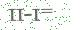Excel中八大经典函数组合，帮你整理齐了！

 后台-系统设置-扩展变量-手机广告位-内容正文顶部

Excel中八大经典函数组合，帮你整理齐了！

Excel精英培训excelpx-tete1、 IF  And

【例】如下图所示，在C列设置公式，如果A列值 小于500 且B列值为 未到期 ，则返回”补款“，否则显示为空。

= IF ( AND (A2<500,B2="未到期"),"补款","")2、 Index  +  Match

【例2】如下图所示，要求根据月份和费用项目，查找金额

E10公式：

= INDEX ( B2:G6, MATCH (B10,\$A\$2:\$A\$6,0), MATCH (A10,\$B\$1:\$G\$1,0) )

= MATCH (B10,\$A\$2:\$A\$6,0)

= MATCH (A10,\$B\$1:\$G\$1,0)

= INDEX(区域,行数,列数）3、 Iferror  Vlookup

【例】如下图所示，要求根据产品名称在上表中查找单价，如果产品不存在则显示为空白

B9公式：

= IFERROR ( VLOOKUP (A8,\$A\$1:\$D\$5,3,0) , "" )4、 Mid  +  Find

【例】在个人信里截取出年龄

B2公式

= MID (A2, FIND (" ",A2)+1,9)5、 Left  +  lenB  +  Len

= LEFT (A2, LENB (A2)- LEN (A2))6、 index  +  Small  Row

【例】如下图所示，要求在F列查找“张明城”的个人消费记录F2公式

{= INDEX (C:C, SMALL (IF(B\$2:B\$10=F\$1, ROW (\$2:\$10)),ROW(A1)))}

• IF(B\$2:B\$10=F\$1,ROW(\$2:\$10)) 如果B列的姓名和F1的姓名相同，就返回它的行号。不相同的返回FALSE

•  Row(a1) ：是返回A1的行号1，如果向下复制会变为 Row(a2)，返回2，其实用它的目的是当公式向下复制时可以生成序号：1，2，3...然后取符合条件的第1个行号，第2个行号...

• SMALL() : 从符合条件的行号中从小到大，逐个提取符合条件的行

• INDEX()  :根据取得的行号从C列提取值

• { } ：数组公式（含有逐一运算的公式）需要按ctrl+shift+enter 输入大括号（一定要是自动生成的，不能手输入大括号）。

7、 Sum   +  Offset  Count

【例】在D2单元格返回B列最近7天的销量

D2公式：

= SUM ( OFFSET (B1, COUNTA(B:B) -7,0,7,1))8、 Sumproduct  Countif

【例】：统计B列的客户数量

= SUMPRODUCT (1/ COUNTIF (B2:B19,B2:B19))工作中最常用的Excel函数公式，全印在一张超大的 鼠标垫 上( 送40集配套视频 )， 点我查看详情

 后台-系统设置-扩展变量-手机广告位-内容正文底部
 留言与评论（共有 0 条评论）

 验证码：